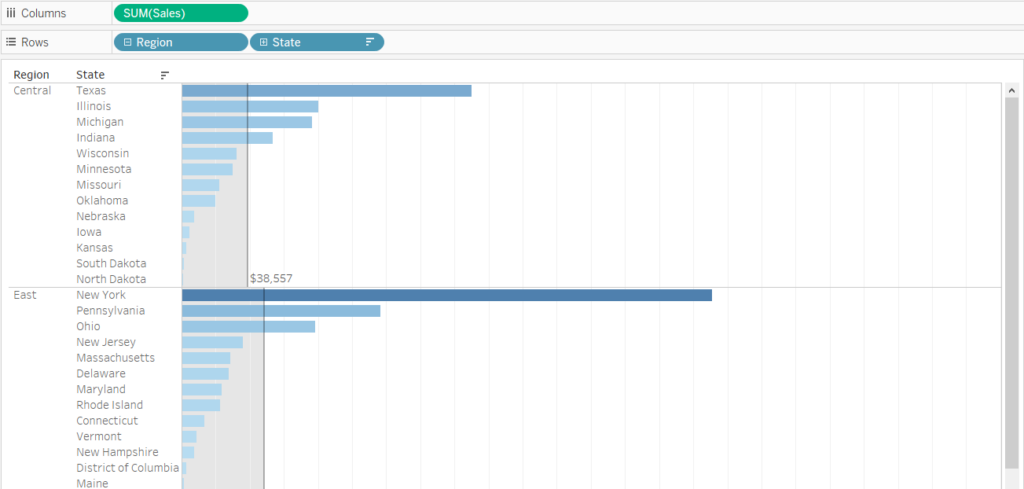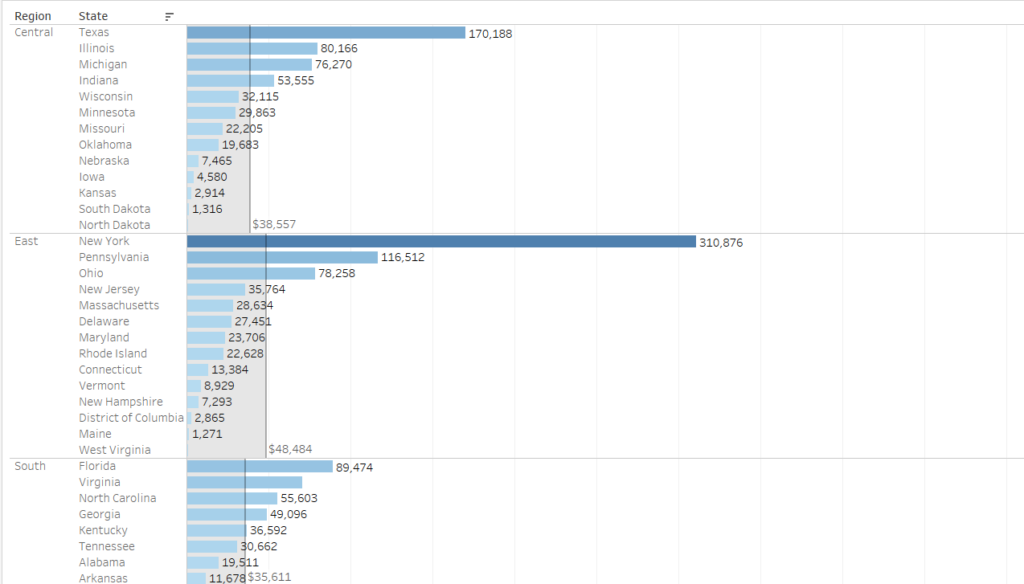## Turning Reference Lines into Calculations: Using window functions and how they can add context to your visualisations

by Owen Barnes

Today we covered a vast amount of Tableau content, and one thing that I finally managed to get my head around was the use of ‘Window’ functions. They were described as ‘a function to show anything within the view’. Window functions are the default function used by Table Calculations, and you can edit how you want to use the function within the ‘table calculation’ configurations.

Lets look at an example with Superstore:Sales by Region and State, with an average line showing the average for each State.

To get this reference line, we simply drag a reference line from the analytics shelf, and drag it onto pane. We choose pane because we want to calculate the average for each individual pane (in this case, Region).

Then, we can edit the reference line, set it to sum(Sales), Average which will give us an average of the sum of sales for each Region. I decided to ‘fill below’ with a light grey colour, and further change the label to show the value of the average in each region.

## Turning this into a function?

If we want to create a calculation out of this, we can use WINDOW_AVG to help us. Setting up the calculation is as follows:

Window_Avg(sum([Sales]))

Remember, we need to aggregate whatever is inside of a Window function. To remember this, think of how you create a table calculation from the Rows or Columns card; the data is already aggregated (likely using sum)!

What this calculation is saying is, ‘calculate the sum of sales, and give me the average of this value’. If we drag it into the view without configuring the table calculation scope, we simple get the sum of sales for each individual state. This is because we have not set up the scope of the table calculation properly.Here, I dragged my calculated field onto labels, and we can see we just get the sum of sales on each bar (State).

So, we need to configure the calculation so that for each region, it returns the average of the sum of all of the State’s sales. For example, for the Central Region, we want to see \$38,557 (as per the reference line).

To do this, we want our calculation to read ‘calculate the sum of sales, and give me the average of this value for every individual region’.

To set this up, we need to configure our table calculation:

So, it looks a bit confusing – why do I untick region if i want to group by it? The honest answer is I don’t know, and it’s very frustrating and counter-intuitive to untick something you want to group by.

However, if you remember to untick what you are trying to group by, over time it will become easier to remember. Here is a nice image of how to read table calculation configurations!

This image really helped me. Since we wrote our table calculation in a calculated field, the calculation type will not show up, but it is ‘Average’ in this case. So we may read this as

1. For each Region
2. Compute the Average of the Total Sales by State

Now we have this correctly computed, we may want to colour our bars depending on whether they are over or under the average sales in that region. To set this up, simply create a boolean field like so:

sum(Sales) > [<Name of your Window_Avg Field Name>]

In my case:

sum(Sales) > [Window Avg Sales/Region]

Drag this onto colour, remove the reference line, and the calculation from the text, and you should have something like this!

This is a great way to add some context to your bar chart, and I think it will help draw some attention to the states that are either doing well, or poorly (however you decide to look at it!)

I hope this helps you when using window functions within Tableau. I was unable to find any examples of use cases when I stumbled across them, but now their use makes much more sense!Owen Barnes

Fri 08 Jan 2021

Tue 05 Jan 2021

Mon 28 Dec 2020

Wed 09 Dec 2020